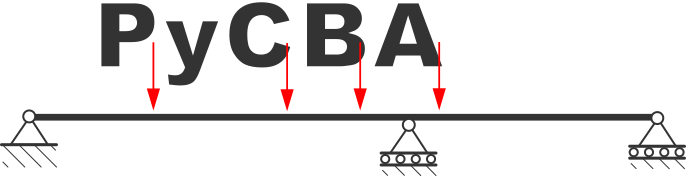# PyCBA – Python Continuous Beam Analysis`PyCBA` is a python implementation of the Continuous Beam Analysis program, originally coded in Matlab and subsequently ported to C++ (by Pierrot).

`PyCBA` is for fast linear elastic analysis of general beam configurations. It uses the matrix stiffness method to determine the displacements at each node. These are then used to determine the member end forces. Exact expressions are then used to determine the distribution of shear, moment, and rotation along each member. Cumulative trapezoidal integration is then used to determine the rotations and deflections along each member. The program features:

One of the main functions of `PyCBA` is that the basic analysis engine forms the basis for higher-level analysis. Current `PyCBA` includes modules for: